Advice: Picking out members of a solution set

When Maple's various "solve" commands (including solve , dsolve , fsolve , isolve , msolve and rsolve ) return the values of more than one variable or function, they generally do so in the form of a set of equations, e.g.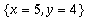. As with any set, the order in which these occur is unpredictable. In particular, if you save your worksheet and reload it in another session, the answer that wasthe first time may well be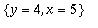the next time. Therefore it is dangerous to try to extract the parts of a solution in a way that depends on the order in which they are presented in the set. Instead, the recommended method is to use the subs command.

In the case of the numerical solution of a differential equation ( dsolve(...,numeric) ), the default is to return a procedure whose output will be a list of values of the dependent and independent variables. You can then use subs to extract the value of the variable you want. On the other hand, dsolve(..., numeric, output=listprocedure) returns a list from which subs extracts a procedure to calculate the value of each variable. Alternatively, dsolve(..., numeric, values=...) returns a matrix with the values of the dependent variables at each of an array of values of the independent variable.

Examples:

> solution:= solve({ x + y = 9, x - y = 1},{x,y});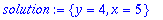> xvalue:= subs(solution,x);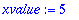> xy:= subs(solution,[x,y]);> q:= subs(solution, x^2 + y^2);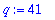> de:= {D(x)(t)=x(t)+y(t), D(y)(t)=y(t), x(0)=1, y(0)=1}:

> desol1:= dsolve(de, [x(t),y(t)]);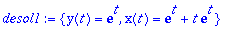> x1:= unapply(subs(desol1,x(t)), t);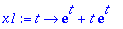> desol2:= dsolve(de, [x(t),y(t)],numeric);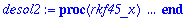> desol2(1);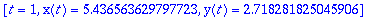> subs(", x(t));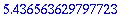> desol3:=dsolve(de,[x(t),y(t)],numeric, output=listprocedure);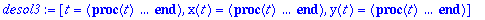> x3:= subs(lsol,x(t));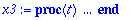> x3(1);> desol4:= dsolve(de,[x(t),y(t)],numeric, value=array([0,1,2]));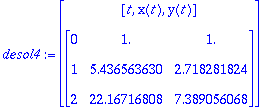See also: subs , solve , dsolve , dsolve/numeric , fsolve , isolve , msolve , rsolve

Maple Advisor Database, R. Israel 1997